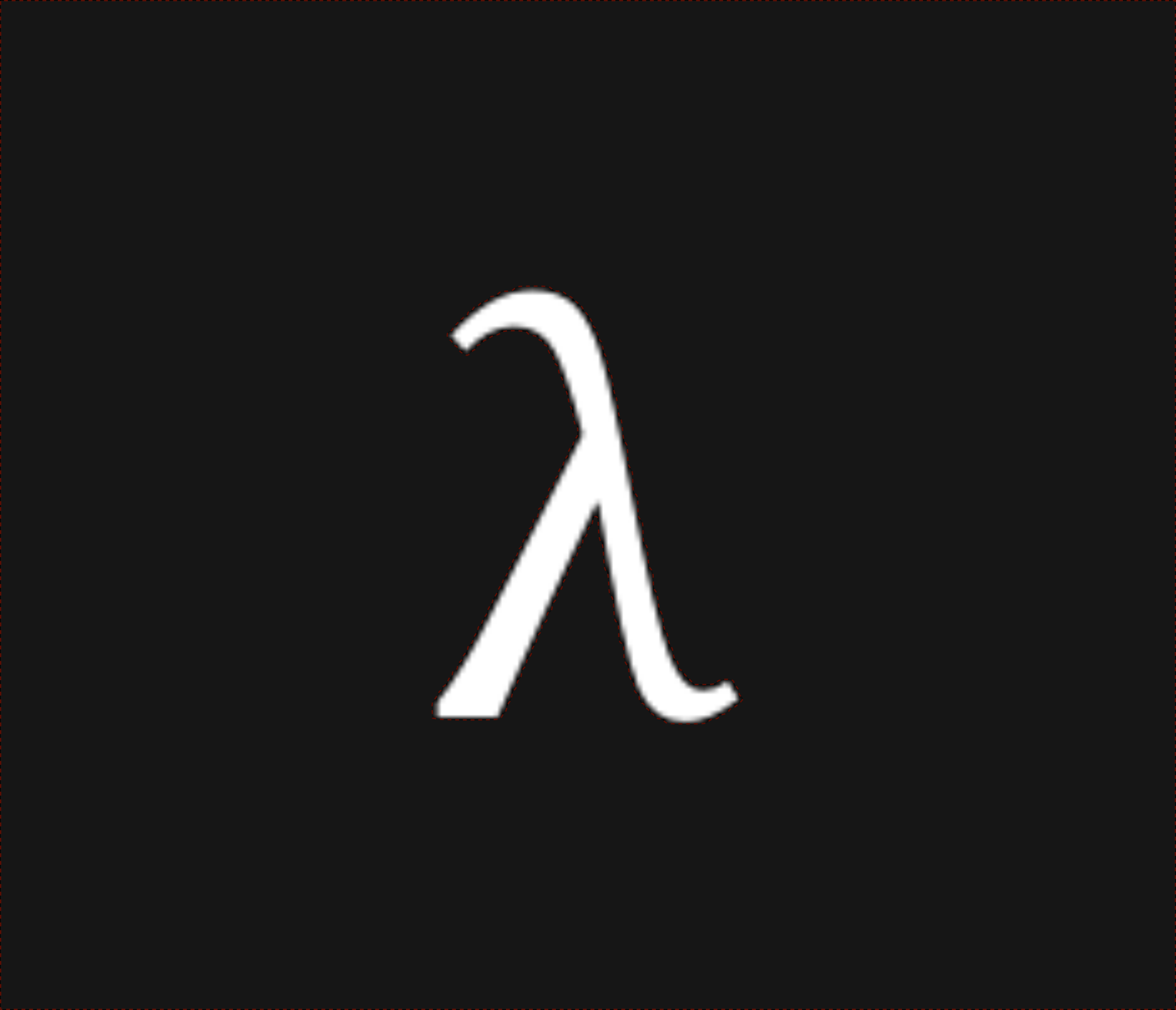nixpulvis

## Achilles' Tent Notes #13 written by Nathan Lilienthal on December 13, 2019.

### Party Perspectives @P { ... }

When writing classic local programs (e.g. Rust 2018) all bindings have affinity to the only local party, you (or @me). To bridge the gap into the MPC context, we allow global! blocks @P { e } to only evaluate into Some(e) if the party running the block locally is @P.

// Ideal syntax?
let r: i32@A = @A { rand() };

// Macro syntax? Closer to what exists today.
let r: Option<i32> = global!(@A, rand());


This composes with conceal which can accept an Option<T> from a party.

let s: Obliv<i32> = conceal!(@P <= @A, r);


conceal! also gives shorthand for creating and consuming the Option at once.

let s: Obliv<i32> = conceal!(@P <= @A { rand() });


The following would fail to type check, since r is only Some for @A, but we’re saying here that @B is providing this value. This implies that Option<i32> really isn’t going to work, we need i32@P, which acts like an optional.

let s: Obliv<i32> = conceal!(@P <= @B, r);


To participate in a protocol where we do not know the block which the other party is executing, we can use concealed!(@P <= @B), which is shorthand for conceal!(@P <= @B, None).

let s: Obliv<i32> = concealed!(@P <= @B);


This allows @A and @B to write partially separated code. For example below, @A calls rand for s, and @B calls prompt (perhaps reading from STDIN) for t, without each other knowing about how these binding’s values were generated.

// @A's code:
let s = conceal!(@P <= @A { rand() });
let t = concealed!(@P <= @B);

// @B's code:
let s = concealed!(@P <= @A);
let t = conceal!(@P <= @B { prompt() });


Which could be rewritten to expose the rand and read calls globally as a single source program once more.

let s = conceal!(@P <= @A { rand() });
let t = conceal!(@P <= @B { prompt() });


Finally, we want to reveal some information from @P to another party @A.

let o: i32@A = reveal!(@P => @A, s + t);


### Special @me Party

TODO: More thought needs to be given to where @P should show up in this example below. I’m starting to wonder if it’s something that belongs on the reference, related to the lifetimes.

fn is_mine<@P, 'a, T>(x: &'a T @P) -> bool {
if @P == @me {
true
} else {
false
}
}

// Always true.
let x = 1;
assert!(is_mine(&x));

// Fails for @B.
let x = @A { 1 };
assert!(is_mine(&x));


### Example invertPerm Function

// TODO: Purpose statements for the four helper functions.
fn randomPermutation() -> Vec<usize>;
fn invertPermutation(v: &[usize]) -> Vec<usize>;
fn waksmanBits(v: Vec<usize>) -> Vec<usize>;
fn waksmanPerm(v: Vec<Obliv<usize>>, w: Vec<Obliv<usize>>)
-> Vec<Obliv<usize>>;

fn invertPerm<@P, @A, @B>(x: Vec<Obliv<usize>>)
-> Vec<Obliv<usize>>
where @P: @A + @B
{
let (pa, pa_waksman) = conceal!(@P <= @A {
let lpa: Vec<usize> = randomPermutation();
let lpa_waksman: Vec<usize> = waksmansBits(&lpa);
(lpa, lpa_waksman)
});

let pb: Vec<Obliv<usize>> = waksmanPerm(&x, &pa_waksman);
let pb_local: Vec<usize@B> = pb.map(|e| reveal!(e, @P => @B)).collect();

let pbinv_waksman = conceal!(@P <= @B {
let pbinv: Vec<usize> = invertPermutation(&pb_local);
waksmanBits(&pbinv)
});

waksmanPerm(&pa, &pbinv_waksman)
}